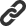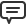# Blog

All Blog Posts  |  Next Post  |  Previous Post

#TMS FMX Chart update with new virtual mode available

Bookmarks:

#### Thursday, October 22, 2015

Where in earlier versions, the method for adding values to a chart was via multiple calls to TMSFMXChart.Series[x].AddSinglePoint(), the new virtual mode offers a faster solution that is more flexible and typically consumes less memory. This can of course make a welcome difference when creating applications for mobile devices.
To start using the new TMS FMX Chart virtual mode, two events need to be implemented:

TMSFMXChart.OnGetNumberOfPoints()
and
TMSFMXChart.OnGetPoint()

In the first event, OnGetNumberOfPoints(), that is triggered for each series in the chart, the number of desired data points can be returned via the ANumberOfPoints parameter.
In this sample event handler, the number of points is set to 10000 for each series in the chart:
```procedure TForm1.TMSFMXChart1GetNumberOfPoints(Sender: TObject;
ASerie: TTMSFMXChartSerie; var ANumberOfPoints: Integer);
begin
ANumberOfPoints := 10000;
end;
```
The second event, OnGetPoint() is used to return the value for each point in the chart series. Here comes the flexibility that these values can be retrieved directly from another data structure (even a database). In this sample example, we assume the values are in a dynamic array of 10000 values and for the sake of this example filled with random floating point values. This is the code that initializes two dynamic arrays that have the data for 2 series:
```var
data_arr_1, data_arr_2: array of double;
begin
SetLength(data_arr_1,10000);
SetLength(data_arr_2,10000);
for i := 0 to Length(data_arr) - 1 do
begin
data_arr_1[i] := random(100) / 33;
data_arr_2[i] := random(200) / 25;
end;
end;```
and now the event handler that sets the values for the virtual chart:
```procedure TForm1.TMSFMXChart1GetPoint(Sender: TObject;
ASerie: TTMSFMXChartSerie; AIndex: Integer;
var APoint: TTMSFMXChartPointVirtual);
begin
case ASerie.Index of
0: if AIndex < Length(data_arr_1) then
APoint.YValue  := data_arr_1[AIndex]
1: if AIndex < Length(data_arr_2) then
APoint.YValue  := data_arr_2[AIndex]
end;
end;
```
A final touch is to apply some settings that will let the Y-axis scale automatically adapt to the values in the series and define the number of visible points along the X-axis (in this case 100 points of 10000 simultaneously visible along the X-axis, other values are shown when the chart is horizontally scrolled). This is done with:
```  TMSFMXChart1.Series.AutoYRange := arEnabled;
TMSFMXChart1.Series.MaxX := 100;
TMSFMXChart1.Series.AutoYRange := arEnabled;
TMSFMXChart1.Series.MaxX := 100;
```

Bruno Fierens

Bookmarks:#### This blog post has received 1 comment.1. Thursday, October 22, 2015 at 7:23:02 PM

That''s clever move, especially when one wants to attach custom data containers to the chart.

Gromov Vsevolod